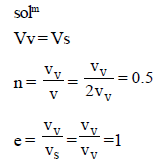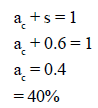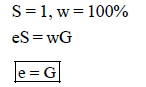Test: Soil Mechanics - 1

# Test: Soil Mechanics - 1

Test Description

## 25 Questions MCQ Test Civil Engineering SSC JE (Technical) | Test: Soil Mechanics - 1

Test: Soil Mechanics - 1 for Civil Engineering (CE) 2023 is part of Civil Engineering SSC JE (Technical) preparation. The Test: Soil Mechanics - 1 questions and answers have been prepared according to the Civil Engineering (CE) exam syllabus.The Test: Soil Mechanics - 1 MCQs are made for Civil Engineering (CE) 2023 Exam. Find important definitions, questions, notes, meanings, examples, exercises, MCQs and online tests for Test: Soil Mechanics - 1 below.
Solutions of Test: Soil Mechanics - 1 questions in English are available as part of our Civil Engineering SSC JE (Technical) for Civil Engineering (CE) & Test: Soil Mechanics - 1 solutions in Hindi for Civil Engineering SSC JE (Technical) course. Download more important topics, notes, lectures and mock test series for Civil Engineering (CE) Exam by signing up for free. Attempt Test: Soil Mechanics - 1 | 25 questions in 50 minutes | Mock test for Civil Engineering (CE) preparation | Free important questions MCQ to study Civil Engineering SSC JE (Technical) for Civil Engineering (CE) Exam | Download free PDF with solutions
 1 Crore+ students have signed up on EduRev. Have you?
Test: Soil Mechanics - 1 - Question 1

### Which of the following types of soil is transported by gravitational forces?

Test: Soil Mechanics - 1 - Question 2

### Water content of soil can

Detailed Solution for Test: Soil Mechanics - 1 - Question 2

In soil Mechanics Water Content (w) = Weight of water/weight of Soil.

take 0.9 Kg of dry Soil and add water about 1 liter (in volume) which is about 1Kg in weight.

Then the water content of mixture = 1/0.9 = 1.11 = 111%

which is greater than 100%,

Mud or slurry tends to have water content more than 100%.

Test: Soil Mechanics - 1 - Question 3

### Which of the following types of soil is transported by gravitational forces?

Test: Soil Mechanics - 1 - Question 4

A fully saturated soil is said to be

Test: Soil Mechanics - 1 - Question 5

Total number of stress components at a point within a soil mass loaded at its boundary is

Detailed Solution for Test: Soil Mechanics - 1 - Question 5

Explanation : Considering a cube will give us 6 stresses till where it’s easy to get. And rest counting as 3 shear stresses along each plane in each direction will give total 9 stresses.

Test: Soil Mechanics - 1 - Question 6

The submerged density of soil in terms of unit weight of watergw, specific gravity G and Voids
ratio e is given by the expression

Test: Soil Mechanics - 1 - Question 7

A soil has a bulk density of 22 kN/m3 and water content 10%. The dry density of soil is

Test: Soil Mechanics - 1 - Question 8

If the voids of a soil mass are full of air only, the soil is termed as

Test: Soil Mechanics - 1 - Question 9

Valid range for n, the percentage voids, is

Test: Soil Mechanics - 1 - Question 10

Select the correct statement.

Test: Soil Mechanics - 1 - Question 11

Voids ratio of a soil mass can

Detailed Solution for Test: Soil Mechanics - 1 - Question 11

Explanation : The void ratio is the ratio of the volume of voids (open spaces, i.e. air and water) in a soil to volume of solids. The void ratio is thus a ratio which can be greater than 1. It can also be expressed as a fraction. Void ratio and porosity differ only in the denominator.

Test: Soil Mechanics - 1 - Question 12

If the volume of voids is equal to the volume of solids in a soil mass, then the values of porosity and voids ratio respectively are

Detailed Solution for Test: Soil Mechanics - 1 - Question 12Test: Soil Mechanics - 1 - Question 13

When the degree of saturation is zero, the soil mass under consideration represents

Test: Soil Mechanics - 1 - Question 14

In a liquid limit test, the moisture content at 10 blows was 70% and that at 100 blows was 20%. The liquid limit of the soil, is

Detailed Solution for Test: Soil Mechanics - 1 - Question 14

The liquid limit of the soil is 65 %

• Liquid limit is define as water content at which the soil possess an arbitrarily fixed small amount of shear strength.
• The result of test are represented in form of curve known as flow curve from which water content corresponding to 25 no. of blows is interpolated and is called as liquid limit.
• Graph is shown in the attached figure.

Let N be the number of blows and w be the water content at N blows.

Liquid limit = w × (N/25)⁰°¹²¹

For 10 number of blows = w (l) = 70 (10/25)⁰°¹²¹ = 89.55 %

For 100 number of blows  = 20 ( 100/25)⁰°¹²¹ = 23.65 %

So liquid limit is = 89.55 % - 23.65 % ≈ 65 %

Test: Soil Mechanics - 1 - Question 15

If the degree of saturated of a partially saturated soil is 60% then air content of the soil is

Detailed Solution for Test: Soil Mechanics - 1 - Question 15Test: Soil Mechanics - 1 - Question 16

If the water content of a fully, saturated soil mass is 100%, then the voids ratio of the sample is

Detailed Solution for Test: Soil Mechanics - 1 - Question 16Test: Soil Mechanics - 1 - Question 17

The ratio of the volume of voids to the volume of soil solids in a given soil mass, is known

Test: Soil Mechanics - 1 - Question 18

Relative density of a compacted dense sand is approximately equal to

Test: Soil Mechanics - 1 - Question 19

If the sand in - situ is in its densest state, then the relative density of sand is

Test: Soil Mechanics - 1 - Question 20

Which of the following methods is most accurate for the determination of the water content of soil?

Test: Soil Mechanics - 1 - Question 21

For proper field control which of the following methods is best suited for quick determination of water content of a soil mass?

Test: Soil Mechanics - 1 - Question 22

A pycnometer is used to determine

Test: Soil Mechanics - 1 - Question 23

Stoke’s law is valid only if the size of particle is

Test: Soil Mechanics - 1 - Question 24

In hydrometer analysis for a soil mass

Test: Soil Mechanics - 1 - Question 25

The hydrometer method of sedimentation analysis differs from the pipette analysis mainly in

## Civil Engineering SSC JE (Technical)

2 videos|122 docs|50 tests
 Use Code STAYHOME200 and get INR 200 additional OFF Use Coupon Code
Information about Test: Soil Mechanics - 1 Page
In this test you can find the Exam questions for Test: Soil Mechanics - 1 solved & explained in the simplest way possible. Besides giving Questions and answers for Test: Soil Mechanics - 1, EduRev gives you an ample number of Online tests for practice

## Civil Engineering SSC JE (Technical)

2 videos|122 docs|50 tests